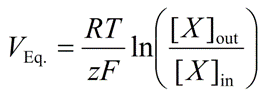PhysiologyWeb  Home  |  FAQ  |  About  |  Contact  |   - Sign InNernst Equation Calculator
The Nernst equation calculates the equilibrium potential (also referred to as the Nernst potential) for an ion based on the charge on the ion (i.e., its valence) and its concentration gradient across the membrane. Temperature also influences the Nernst potential (see Nernst equation below). A Nernst potential will develop across a membrane if two criteria are met: (1) if a concentration gradient exists across the membrane for a given ion, and (2) if selective permeation pathways (i.e., selective ion channels) exist that allow transmembrane movement of the ion of interest. Finally, for selective ion channels, where the selectivity filter strongly favors the permeation of one ion over other ions, the Nernst potential also predicts the reversal potential (Vrev) of the current-voltage (I-V) relationship.
The Nernst equation• VEq. is the equilibrium potential (Nernst potential) for a given ion. It is common to use the ion symbol as a subscript to denote the equilibrium potential for that ion (e.g., VK, VNa, VCl, VCa, etc.). If only one ionic species is present in the system, and/or channels for only one ionic species are present (and open), then VEq. will also be the membrane potential (Vm). Note that the unit of VEq. is the Volt. However, the equilibrium potential is typically reported in millivolts (mV). If two or more ions contribute to the membrane potential, the Nernst potential no longer yields the Vm. In this case, use the Goldman-Hodgkin-Katz (GHK) equation to calculate the Vm.
• R is the universal gas constant and is equal to 8.314 J.K-1.mol-1 (Joules per Kelvin per mole).
• T is the temperature in Kelvin (K = °C + 273.15).
• z is the valence of the ionic species. For example, z is +1 for Na+, +1 for K+, +2 for Ca2+, -1 for Cl-, etc. Note that z is unitless.
• F is the Faraday's constant and is equal to 96485 C.mol-1 (Coulombs per mole).
• [X]out is the concentration of the ionic species X in the extracellular fluid. Note that the concentration unit must match that of [X]in.
• [X]in is the concentration of the ionic species X in the intracellular fluid. Note that the concentration unit must match that of [X]out. Typically, but not always, the concentrations are noted in mM.
• The Nernst equation can be derived based on simple thermodynamic principles (see Derivation of the Nernst Equation).
Constant terms in the Nernst equation
• Universal Gas Constant (R) = 8.314 J.K-1.mol-1 (Joules per Kelvin per mole)
• Faraday's Constant (F) = 96485 C.mol-1 (Coulombs per mole)
Nernst equation calculator
Each calculator cell shown below corresponds to a term in the formula presented above. Enter appropriate values in all cells except the one you wish to calculate. Therefore, at least four cells must have values, and no more than one cell may be blank. The value of the blank cell will be calculated based on the other values entered. After a calculation is performed, the calculated cell will be highlighted and subsequent calculations will calculate the value of the highlighted cell (with no requirement to have a blank cell). However, a blank cell has priority over a highlighted cell.
Please note that the unit of temperature used in the Nernst equation is the Kelvin. It is also important to note that although this worksheet allows you to select different concentration units, during the calculation, the numerator and denominator units are converted so that they match. This ensures that the fraction ([X]o/[X]i) will be unitless. Also note that based on the constants used (R = 3.814 J.K-1.mol-1 and F = 96485 C.mol-1), the unit of VEq. will be in Volts. Keeping this fact in mind, this tool simplifies the calculation by allowing you to calculate directly to or from mV.
 T : K z : [X]out : pM     nM     μM     mM     M [X]in : pM     nM     μM     mM     M VEq. : mV     V

Interpretation of the Nernst potential
As mentioned above, the Nernst equation calculates the equilibrium potential (VEq.) for an ion based on the charge on the ion (i.e., its valence) and its concentration gradient across the membrane. If only one ionic species is present in the system and/or only one type of ion channel is present, the Nernst potential also determines the resulting membrane potential (Vm). Of course, it must be assumed that the ion channels are open in order to allow transmembrane movement of the ionic species for which the channel is specific. If channels for two or more ions are present (and are open), both ions contribute to the membrane potential. In this case, Vm will not be at the equilibrium potential for either ion and, thus, no ion will be at equilibrium. Instead, the value of Vm will be determined by the relative contribution of each ion to the membrane potential. The relative contribution of each ion is governed not only by its concentration gradient and valence, but also by its relative permeability. Membrane permeability for an ion is defined as the relative ease with which the ion crosses the membrane, and is proportional to the total number of open channels for that ion in the membrane. To calculate the membrane potential when two or more ions play a role, use the Goldman-Hodgkin-Katz (GHK) equation.

Posted: Tuesday, December 20, 2005
Last updated: Sunday, June 12, 2011